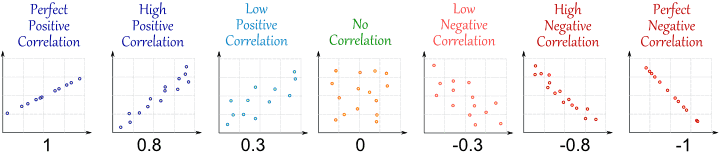# Correlation

Correlation: A statistical measure used to see how one continuous variable changes with respect to another continuous variable.

It yields a “correlation coefficient”.

Correlation coefficient: A statistical measure of the relationship between two continuous variables. It ranges from -1 to +1.A set of figures showing the levels of correlation

A correlation coefficient of -1 indicates perfect negative correlation, while a correlation coefficient of +1 indicates perfect positive correlation. A correlation coefficient of 0 (zero) indicates no correlation.

Positive correlation: When the values of one continuous variable increase, the values of the other continuous variable also increase.

Example: The relationship between age and height- as age increases, height also increases.

Negative correlation: When the values of one continuous variable increase, the values of the other continuous variable decrease.

Example: The relationship between Body Mass Index (BMI) and running speed- as BMI increases, running speed decreases.

No correlation: When the values of one continuous variable increase, there is no influence on the values of the other continuous variable, either positive, or negative. There is no relationship between the two continuous variables.

Example: The relationship between scores of corrupt officials in a test on anti-corruption laws, and the number of corruption-related offences committed by the same officers. The test scores are unlikely to have any relationship with actual practice.A set of figures illustrating positive, zero and negative correlation

The above image illustrates the correlation using a set of examples from the automotive industry.

We see that as the mileage (number of miles traveled) of the vehicle increases, its value decreases. This is an example of negative correlation.

The second figure shows the relationship between the quality of a vehicle and its color- none. This is an example of zero correlation.

The last figure shows the relationship between the number of accidents and cost of vehicle insurance- as the number of accidents increases, so does the cost of insurance. This is an example of positive correlation.